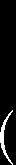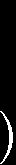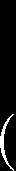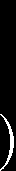## Microsoft word - limassol_revised.docx

Recovery of a Lattice Generator Matrix from its Gram Matrix for
Feedback and Precoding in MIMO
Francisco A. Monteiro, Student Member, IEEE, and Ian J. Wassell
Abstract— Either in communication or in control applications,
coding, MIMO spatial multiplexing, and even OFDM) multiple-input multiple-output systems often assume the
unified from a lattice perspective as a general equalization knowledge of a matrix that relates the input and output vectors.
problem (e.g., ). Advances in lattice theory are therefore For discrete inputs, this linear transformation generates a
multidimensional lattice. The same lattice may be described by
an infinite number of generator matrixes, even if the rotated
There are several ways of describing a lattice (e.g., via versions of a lattice are not considered. While obtaining the
modular equations  or trellis structures ), however, Gram matrix from a given generator matrix is a trivial
the two most popular ones in engineering applications are i) operation, the converse is not obvious for non-square matrixes
the generator matrix and ii) the Gram matrix. The and is a research topic in algorithmic number theory. This
computation of the latter given the former is trivial. The paper proposes a method to execute such a conversion and
reverse is not, and an efficient algorithm for this conversion applies it in a novel MIMO system implementation where some
remains an open problem in the theory of lattices. of the complexity is taken from the receiver to the transmitter.
It should be noticed that an efficient algorithm for this Additionally, given the symmetry of the Gram matrix, the
reverse operation can allow a lattice to be described using number of elements required in the feedback channel is nearly
vectors where each corresponds to the ith column of the n×n generator matrix H (considering full-rank lattices only).
Consequently, one can state that G induces a quadratic
Each integer xi is an element of the column vectors x. Thus, form and is definite positive because
a lattice defined as in (1) is the span of the column space of This permits us to say that always has a LDLT
the generator matrix H, when we restrict the input to integers. decomposition .
Note that the prevalent notation in MIMO literature Obtaining a valid from is not simple. defines an considers column vectors while in channel coding or in other abstract lattice, however, two versions of a lattice will have fields in mathematics lattices are traditionally represented by the same Gram matrix and in general, for a given , the span of the row space of a generator matrix. Notice that rows and columns of a given H span different lattices.
can be transformed into one factorization problem. When representing an equivalent lattice defined by decomposition offers a good solution as it applies to symmetric definite positive matrices . For the general m×n case, obtaining a basis from a specified Gram matrix had no available method in the literature until recently . is an unitary matrix (with real elements and is a unimodular matrix (with C. The volume of lattices Full rank lattices are specified by a full-rank generator matrix and the volume of a lattice (e.g., ) is given by With this in mind, the unitary transformation performs a rigid rotation of the lattice structure (i.e., of its generating vectors), while the unimodular matrix replaces a set of is rank-deficient (which is the case when generating vectors by a different set that still generates the non-square), the volume is One of the hardest lattice problems is the lattice distinguishing problem, i.e., to discover if two lattices are For a traditional complex representation with N and NR antennas as outputs, the received signal is question becomes trivial. But when both transformations are unknown the question is difficult to solve in some particular problems ,. This decision problem has a simple solution when the lattices are rational lattices (when all entries are in ). In that case two lattices are equivalent if and only if their generating matrixes have the same Hermite Normal Form ,. However, the real lattices that arise mean circularly symmetric Gaussian random variable with in communication problems lead to numerical problems given the large numerators and denominators in the fractions unitary variance. The noise vector is representing fading coefficients. In that case the best approach is to use the fact that the QR decomposition is with independent circularly symmetric Gaussian unique up to signs in the main diagonal. random variables, each one with a certain variance In the remainder of the paper we will resort to the equivalent real model of complex lattices: where H is the Hermitian operator (conjugate and transpose). between all generating vectors and thus is unique to a lattice subject to unitary transformations (albeit not unique for unimodular transformations should be noticed that, by construction, symmetric (because the inner product is commutative) and a where L is a rational n×n lower triangular matrix with ones
definite positive matrix. This second property can be verified in the diagonal (i.e., is a unit matrix) and D is a n×n diagonal
from the squared Euclidean norm of y (using the Hermitian matrix with rational diagonal entries
Presented at the Int. Symposium on Communications, Control and Signal Processing, Limassol, Cyprus, March 2010. (IEEE Signal Processing Society.) number of squares of R rational numbers, that is, Using traditional singular value decomposition (SVD) An exact expansion of these djj can be accomplished by applying a naive greedy algorithm as proposed for the first time by Lenstra in . Imposing an exact expansion for each often leads to a large number of terms in the sum where is a diagonal matrix and (10) and for that reason Lenstra also proposed the use of a matrices (i.e., norm-preserving rotations, and therefore randomized algorithm given in , which assures the preserves the statistics of the noise term). A SVD-based bound scheme implies one SVD decomposition (requiring at least This paper proposes to replace an exact conversion from flops ) at the Rx in addition to matrix by an approximate conversion (leading to a using a fixed complexity algorithm that can be applied in a requiring flops). This technique achieves capacity real time communication system. Based on the results in , through water filling power allocation (according to the we use a simple greedy algorithm for the expansion and singular values) . truncate the number of terms to In  it was shown that the LDLT decomposition
truncated Lenstra algorithm. One way of achieving this is by achieves better performance than standard SVD, while being using R equal terms in (10). One may notice that when R=1, slightly less complex. Most importantly, that new approach the algorithm resorts to an approximated Cholesky takes advantage of having a precoding matrix with zero elements. In fact it requires a in the One starts by constructing a tall matrix rows and n columns. For the case with R=4 terms for each of triangular matrix is fedback from the R This paper proposes a technique that achieves the same performance as  while removing most of the complexity from the receiver side to the transmitter side, which is an important feature in scenarios where the transmitter is a base station and the receiver terminal should be made as simple as possible, i.e., by avoiding expensive processing. where each row has one and only one non-zero entry. Now, Remark: as in , this paper is not assessing the one can re-construct the diagonal matrix from using capacity-achieving regime and thus, for simplicity, uniform power is allocated to the transmit antennas. Both this proposal and  have a pre-processing stage at We have made this matrix multiplication explicit to Rx consisting of the generation of a definite positive matrix emphasise how this ensures that each djj is a sum of squares G, the Gram matrix of the lattice defined by the columns of
as defined in (10). Finally, the approximated generator . This Gram matrix is formed by the left multiplication (3) In this proposal it is imperative CSIT so that Tx can construct the precoding matrix . This paper shows that his can be achieved with the feedback of a lower triangular matrix only. Given the symmetry of , the Tx only needs to reconstruct the entire matrix, achieving the same bandwidth savings seen in  for the feedback channel. After The complexity of this technique is cubic in the reconstructing the entire (by symmetry), the Tx can use dimension n, due to the LDLT steps, to which we should add the truncated Lenstra algorithm described in Section IV to
for the rational approximation steps. The overall obtain an equivalent generator matrix (Section II) for the , however, as it will be shown lattice. This matrix, later in Table 1, as the LDLT decomposition will be reused, equivalent generator matrix for the lattice, holding the same
only the n term will add to the complexity of the technique Gram matrix. However, it is possible to obtain from them the same and unique generator matrix resulting from QR It should be noticed that, unlike Cholesky decomposition, decompositions remembering that a QR decomposition is this technique is applicable to both square and non-square unique when imposing the positiveness of elements in the matrixes, allowing us to retrieve a rectangular from . Presented at the Int. Symposium on Communications, Control and Signal Processing, Limassol, Cyprus, March 2010. (IEEE Signal Processing Society.)independent transmission channels and ii) geometrically it corresponds to a communication problem over a rectangular lead to and , which would be the same matrix (up signs lattice. Thus, it is convenient to think of a lattice as the result in main diagonal) if there was no distortion associated with of a linear transformation of the cubic lattice in the truncated Lenstra algorithm. A central aspect is that the same Gram matrix is also obtainable from alone, alone or even a mixture of both, given their closeness: orthogonal generating vectors which span a rectangular lattice (this lattice would even have the simplest trellis representation one may have for lattices, e.g., ). The As indicated in Section II.B and in Section IV, would performance increase can be geometrically interpreted from have a LDLT decomposition, which can be calculated not this insight. A rectangular lattice is obtained from a
only given
(in both cases applying (3)) but also deformation of a cubic lattice , or both. However, this will not be the dimension according to each matrix to be LDLT decomposed.
rectangles and thus even a zero-forcing detector experiences Taking advantage of these facts the mode in (7) and be no performance penalty. The right multiplication of the channel matrix by (17) changes the power at the transmitter. The geometric interpretation is also useful on this matter. The “row lattice” The matrixes used in (18) will be presented and justified on the fallowing. First, notice that this made a matrix to appear (a permutation matrix may be needed to have a unique QR), which, despite not being the Gram matrix of the underlying lattice, it is the (approximate) Gram matrix of the lattice spanned by the row Subsequently, one also needs the insertion of a diagonal lattice (as indicated in Section II). This matrix also has an LDLT decomposition and thus (18) can equivalently be scaling
at the precoding stage so that the volume of both lattices underlying the transmission scheme becomes Figure 1 depicts the overall transmission scheme that is proposed while in Figure 2 and in Figure 3 one can observe in detail the processing required respectively at the T Finally, after the detection filter at the receiver, the entire x as well as the fluxes of CSI between both of them. At the Rx it is important to highlight that there are two parallel processing occurring at different stages and each one associated with a different fading block: i) obtain G that will
be sent back to Tx in the form of a triangular matrix and ii) construct the receive filter from a received strictly upper triangular matrix and Q. In fact,
triangular but also a unit lower triangular (ones in the It should be noticed that, as Q is orthogonal (unitary if diagonal). This saves the transmission of the diagonal and
further simplifies the computations at the receiver. The R be forwarded to the Rx. These coefficients are denoted by . The process is summarised in Algorithm 1. (The will then just have to apply the filtering to the channel is assumed to remain unchanged between adjacent incoming precoded signal, i.e., the unavoidable filtering symbols as it is common in the slow fading assumption.) multiplications that are present in all detectors. Besides that, the Rx only needs to compute G and (given its symmetry)
send back to the Tx only the lower or the upper parts of G,
which will be denoted by
are computed and sent from the Tx to the Rx. The resulting transmission chain (20) can be interpreted in two ways: i) algebraically it corresponds to a set of Figure 1: Proposed closed loop transmission scheme. Presented at the Int. Symposium on Communications, Control and Signal Processing, Limassol, Cyprus, March 2010. (IEEE Signal Processing Society.)coefficients flowing in both the uplink and downlink. The number of operations in Table 1 is presented in a way that shows the contribution of each individual processing stage to the total number of operations of the Rx or Tx (matrix complexity at the receiver comes from a QR decomposition and two matrix multiplications: one to initially obtain (similar to ) and then the unavoidable filtering Figure 2: Processing at the transmitter. multiplication by . One should remember that this last multiplication is common to all types of receivers in both To access the approximation one first computes the error matrix of the Gram matrix involved (i.e., the Gram matrix associated with the “row lattice”, as indicated in Section V) 5: Obtain approximate from using the algorithm and one applies to it the squared Frobenius matrix norm  7: Compute the Gram matrix of the “row lattice” at Tx: Figure 4 shows the distribution of this error for three example cases having the number of real dimensions most common in MIMO wireless communications (and with (note: for a non-squared the non-zero rows must be variance 0.5 per real component). Notice that despite the Gram matrix of the “row lattice” 10: Strictly lower triangular matrix is sent: and the one of “column lattice” being different, they hold the statistics and consequently they are interchangeable in (3). (note: can be computed at the same time as steps 4-10) For a NT =4, NR =4 configuration (i.e., n=8 dimensions) under a Rayleigh fading channel and using 16-QAM modulation, it was verified that with the LDLT
multiplies the received decomposition proposed in this paper the error shown in Figure 4 leads to a negligible performance penalty in terms of symbol error rate (SER) in respect to the results presented The number of flops required by the LDLT decomposition in  for the same configuration when using the same
, which is half of the number of flops needed in minimum mean squared error (MMSE) receiver. Gaussian elimination, the number of flops of QR This paper shows how to reconstruct (with very low more efficient algorithms for matrix multiplication). Table 1 distortion and fixed complexity) a generator matrix of a contains a comparison of the proposed technique with SVD lattice from one given Gram matrix of the same lattice for and with  in terms of the number of flops and number of non-square matrixes. This opens new possibilities in several problems of engineering and computer science that rely on Presented at the Int. Symposium on Communications, Control and Signal Processing, Limassol, Cyprus, March 2010. (IEEE Signal Processing Society.) lattice geometry as it breaks through the restriction that the  Daniele Micciancio and Shafi Goldwasser, Complexity of Lattice Cholesky decompositions imposes (valid for squared Problems - A Cryptographic Perspective. Norwell, Massachusetts, matrixes). Subsequently, the paper shows how the algorithm devised in Section IV can be central to a technique for  Ian H. Sloan and Stephen Joe, Lattice Methods for Multiple Integration. Oxford, UK: Oxford University Press, 1994. channel diagonalisation of MIMO systems. With this  J. N. Lyness, "An introduction to lattice rules and their generator technique: i) LDLT decomposition takes place at the transmit
matrices," IMA Journal of Numerical Analysis - Oxford University side; ii) the number of elements to be fedback to T Press, vol. 9, no. 3, pp. 405-419, July 1989. , as in ; iii) the filtering matrix at R  Hendrik W. Lenstra, "Lattices," in Algorithmic Number Theory, J. P. from a unit lower triangular and an orthogonal matrix, which Buhler and P. Stevenhagen, Eds. Cambridge, UK: Cambridge further reduces the complexity of the filtering matrix  John H. Conway and Neil J. A. Sloane, Sphere Packings, Lattices and multiplication at Rx. The extra cost to bear is a QR Groups, 3rd ed. New York, New York, USA: Springer, 1999. decomposition at the Rx. However, a QR module would have  Alexandre Schrijver, Theory of Linear and Integer Programming. to exist at Rx if typical open-loop spatial multiplexing Chischester, UK: John Wiley & Sons, 1986, ch. 4, 5, 6. schemes are also to be supported. For large number of  Wei Zhang, Xiaoli Ma, B. Gestner, and D. Anderson, "Designing low- antennas, the presented closed loop architecture (i.e., with complexity equalizers for wireless systems," IEEE Communications CSIT) for MIMO communications nearly halves the number , vol. 47, no. 1, pp. 56-62, January 2009. of coefficients traditionally needed to represent the channel.  A. Paz and C. P. Schnorr, "Approximating integer lattices by lattices with cycle factor groups," in Proceedings of 14th Int Conf on Automata, Languages and Programming, Karlsruhe, Germany, LNCS  G. David Forney, "Density / length profiles and trellis complexity of lattices," IEEE Transactions on Information Theory, vol. 40, no. 6, pp.  David J. Love, Robert W. Heath, Wiroonsak Santipach, and Michael L. Honig, "What is the value of limited feedback for MIMO channels," IEEE Communications Magazine, vol. 42, no. 10, pp. 54-59, October  Michael Szydlo, "Hypercubic lattice reduction and analysis of GGH and NTRU signatures," in Proceedings of Eurocrypt 2003, Warsaw, Poland, LNCS 2656, Germany, 2003, pp. 433-448.  Erik Agrell, Alexander Vardy Thomas Eriksoon, and Kenneth Zeger, "Closest point in lattices," IEEE Transactions on Information Theory, vol. 48, no. 8, pp. 2201-2214, August 2002.  Robert Piziak and P. L. Odell, Matrix Theory - From Generalized Figure 4: Probability distribution of the squared Frobenius norm of the error Inverses to Jordan Form. Boca Raton, FL: Chapman & Hall - CRC,  G. W. Stewart, Introduction to Matrix Computations. London, UK:  Fernando Pérez-Cruz, Miguel R. Rodrigues, and Sergio Verdú,  Gene H. Golub and Charles F. Van Loan, Matrix Computations, 3rd "Optimal precoding for digital subscriber lines," in Proceedings of ed. Baltimore, Maryland, USA: The Hohns Hopkins University Press, ICC'08 - The IEEE Inter. Conf. on Communications, Beijing, May  M. O. Rabin and J. O. Shallit, "Randomized algorithms in number  Per Ödlig, Thomas Magesacher, Per Ola Börjesson Stefan öst, Miguel theory," Communications on Pure and Applied Mathematics, vol. Berg, and Enrique Areizaga, "The forth generation broadband concept," IEEE Communications Magazine, vol. 43, no. 1, pp. 63-69,  Jürgen Lindner, "Transmission over MIMO channels: on diversiy and spacial multiplexing," in Proceedings of ISCTA´07 - The Inter.  M. Vedat Eyuboglu and G. David Forney, "Lattice and trellis Symposium on Communication Theory and Applications, Ambleside, quantization with lattice- and trellis-bounded codebooks - high-rate theory for memoryless sources," IEEE Transactions on Information  Kenichi Kobayashi, Tomoaki Ohtsuki, and Toshinobu Kaneko, theory, vol. 39, no. 1, pp. 46-58, January 1993. "MIMO Systems in the Presence of Feedback Delay," IEICE  Erik Agrell and Thomas Eriksson, "Optimization of lattices for Transactions on Communications, vol. E91-B, no. 3, pp. 829-836, quantization," IEEE Transactions on Information Theory, vol. 44, no.  Ezio Biglieri and Giorgio Taricco, Transmission and Reception with  Robert M. Gray and David L. Neuhoff, "Quantization," IEEE Transactions on Information theory, vol. 44, no. 6, pp. 2325-2383, Massachusetts, USA: now Publishers, 2004.  Che-Chen Chou, Hsi-Chei Chen, and Jen-Ming Wu, "A low  R. Cortelazzo and G. Manduchi, "On the determinant of all sublattices complexity channel decomposition and feedback strategy for MIMO of preassigned index and its applications to multidimensional precoder design," in Proc. of ICASSP'09 - The IEEE Int. Conf. on sampling," IEEE Transactions on Circuits and Systems for Video Acoustics, Speech and Signal Processing, Taipei, Taiwan, April 2009, Technology, vol. 3, no. 4, pp. 318-320, August 1993.  Daniele Micciancio and Oded Regev, "Lattice-based cryptography," in  Ran Raz, "On the complexity of matrix product," in Proceedings of the Post-Quantum Cryptography, Daniel J. Bernstein, Johannes thiry-fourth annual ACM symposium on Theory of computing, Buchmann, and Erik Dahmen, Eds. Berlin, Germany: Springer, 2009, Montreal, Canada, May 2002, pp. 144-151. Presented at the Int. Symposium on Communications, Control and Signal Processing, Limassol, Cyprus, March 2010. (IEEE Signal Processing Society.)

Source: http://iul.pt/~frmo/ISCCSP_2010_revised.pdf

### Huisarts ¥ 706-def

“Mensen laten nadenken over levenseinde ” “Zorgverleners moeten tijd uittrekken om te luisteren naar wat mensenrond euthanasie vertellen. Dat geldt trouwens voor het hele gebeurenrond gezondheid en ziek zijn. Het gaat ook over patiëntenrechten. De artsis niet degene die weet wat goed is voor de patiënt. Hij maakt enkel keu-zes bespreekbaar en begeleidt ze. Artsen mogen hu

### Microsoft word - linezolid_inf_3 ore_scheda tdm

SCHEDA RACCOLTA CAMPIONI DI PK (AUC) Linezolid infusione 3 ore COGNOME E NOME (o ID): _ _ _ _ _ _ _ _ _ _ _ _ _ _ _ _ _ Data di Nascita: _ _ _ _ _ _ _ _ Peso (Kg): _ _ _ _ _ _ Altezza (cm): _ _ _ _ _ _ Sesso: Maschio  Femmina  Stato flogistico in corso: SI  NO  Medico Responsabile: _ _ _ _ _ _ _ _ _ _ _ _ _ Provette Effettiva Commenti Tera